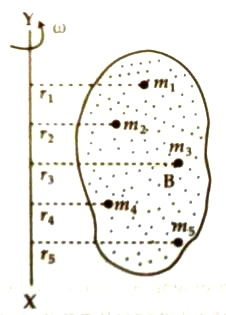# Moment of Inertia

Moment of Inertia is a measure of the resistance of a body to angular acceleration about a given axis that is equal to the sum of the products of each element of mass in the body and the square of the element’s distance from the axis

When a rigid body is confined in a fixed axis, then if force is applied on that body, it cannot move in straight line due to confinement. The body rotates around the axis and there is angular displacement of each of the particles of the body. This type of motion of a body with respect to an axis is called rotational motion. The axis can either be inside or outside the body. If a rigid body rotates around an axis, then moment of inertia of that body with respect to that axis means the summation of the product of square of distance from the axis and mass of each of the particles of that body.Explanation: Let B be a rigid body which is rotating around a fixed axis XY with a uniform angular velocity ω (figure). If the body is the summation of innumerable particles of masses m1, m2, m3… …, mn and the particles are respectively at distances r1, r2, r3, … … …, rn from the exis of rotation, then according to the definition with respect to that axis,

The moment of inertia of first particles = m1r12

The moment of inertia of second particles = m2r22

The moment of inertia of third particles = m3r32 and,

The moment of inertia of forth particles = m4r42

Moment of inertia does not depend on angular velocity of particles, but depends on distribution of particles about the axis of rotation.

Unit and dimension of moment of inertia: In M.K.S. and S.I. system unit of moment of inertia is kilogram-metre (kg-m2) and dimension is [I] = [mass x (distance)2] = [ML2]